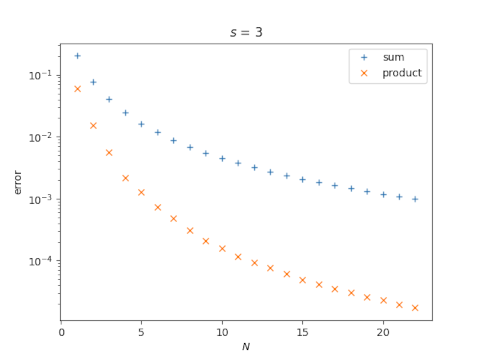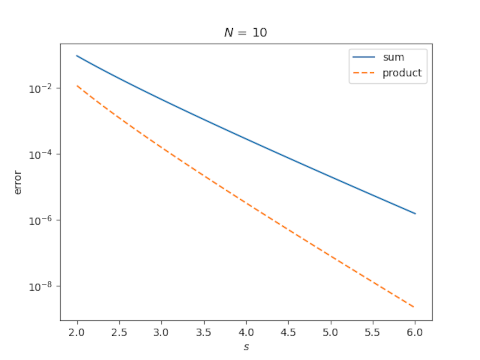# Zeta sum vs zeta product

The Riemann zeta function ζ(s) is given by an infinite sum and an infinite productfor complex numbers s with real part greater than 1 [*].

The infinite sum is equal to the infinite product, but which would give you more accuracy: N terms of the sum or N terms of the product? We’ll take a look at this question empirically.

The accuracy of the partial sum and the partial product depends on N and on s. For starters, let’s fix s = 3 and look at the approximation error as a function of N. Note that the error is plotted on a log scale.Now let’s fix N = 10 and look at the error as a function of s.So our two examples suggest that taking N terms of the product gives more accuracy than taking N terms of the sum.

In the paper mentioned in the previous post, the author approximates the zeta function with a few terms of the product. That’s what motivated this post. I thought the product might give more accuracy than the sum, and that appears to be the case.

## Related posts

[*] If you evaluate the series for ζ at s = -1, despite the fact that the equation does not hold at that point, and evaluate ζ at -1 using analytic continuation, you get the infamous nonsensical result that the positive integers sum to -1/12.

## 2 thoughts on “Zeta sum vs zeta product”

1. GlennF

It’s not too surprising… Even at N = 1, the product is winning because it corresponds to the sum over all multiples of 2, not just the single ‘2’.
Similarly N =2 contains all multiples of 2 and 3 (2^i * 2^j), etc.

2. GlennF

Small correction: I meant multiples of 2 alone (i.e. powers of 2) for N=1